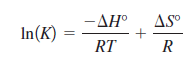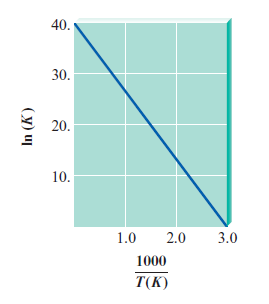# Problem: Consider the relationshipThe equilibrium constant for some hypothetical process was determined as a function of temperature (Kelvin) with the results plotted below.From the plot, determine the values of ΔH° and ΔS° for this process. What would be the major difference in the ln(K) versus 1/T plot for an endothermic process as compared to an exothermic process?

###### FREE Expert Solution

For the first part, we're being asked to determine the values of ΔH° and ΔS° for the process.

We're given the following relationship:

$\overline{){\mathbf{ln}}{\mathbf{\left(}}{\mathbf{K}}{\mathbf{\right)}}{\mathbf{=}}\frac{\mathbf{-}\mathbf{∆}\mathbf{H}\mathbf{°}}{\mathbf{RT}}{\mathbf{+}}\frac{\mathbf{∆}\mathbf{S}\mathbf{°}}{\mathbf{R}}}$

We can interpret the equation as:

b = y-intercept → when x = 0

81% (12 ratings)###### Problem Details

Consider the relationshipThe equilibrium constant for some hypothetical process was determined as a function of temperature (Kelvin) with the results plotted below.From the plot, determine the values of ΔH° and ΔS° for this process. What would be the major difference in the ln(K) versus 1/T plot for an endothermic process as compared to an exothermic process?

Frequently Asked Questions

What scientific concept do you need to know in order to solve this problem?

Our tutors have indicated that to solve this problem you will need to apply the Gibbs Free Energy concept. You can view video lessons to learn Gibbs Free Energy. Or if you need more Gibbs Free Energy practice, you can also practice Gibbs Free Energy practice problems.

What professor is this problem relevant for?

Based on our data, we think this problem is relevant for Professor Delgado's class at FIU.

What textbook is this problem found in?

Our data indicates that this problem or a close variation was asked in Chemistry: An Atoms First Approach - Zumdahl Atoms 1st 2nd Edition. You can also practice Chemistry: An Atoms First Approach - Zumdahl Atoms 1st 2nd Edition practice problems.